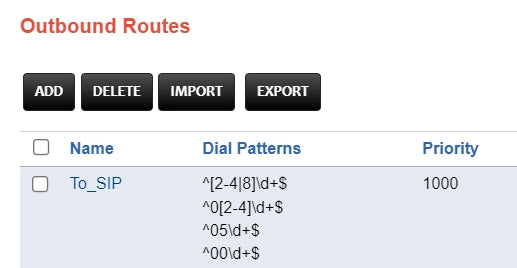# Synway PBX DID Usage

The target number of the incoming call is defined in the DID Number section. To run the accept-all rule in this definition, the DID pattern section can be left blank or ^d+\$ can be written.

The ^ = sign means the beginning of the rule.

d = means any digit between 0 and 9.

+ = means an indefinite number of digits.

The \$ = sign means the end of the rule.

Some examples are as follows;

^d+\$= any number of digits

^[2-4]d+\$ = 02.03 v A number of any length starting with e04

^ [ 2-4|8]d+\$= A number of any length starting with 02,03,04 and 08

^[2-4]dddddd\$= 7 digit number starting with 2,3,4

^05d+\$= A number of any length starting with 05

^00d+\$= A number of any length starting with 00

EXAMPLE; DOMESTIC, OUTSIDE, GSM AND INTERNATIONAL CALL DEFINITIONADDING 0212 AREA CODE IN LOCAL CALLS AND DEFINITION OF OTHER PREFIXES